﻿ Computer transistors do not use quantum mechanics.

# PC doesn't use quantum mechanics.

Summary

## Electronic device is classical mechanics.

### [ The current computer doesn't use quantum mechanics ! ]

(Fig.1)  ↓ Computer CPU uses classical electromagnetism.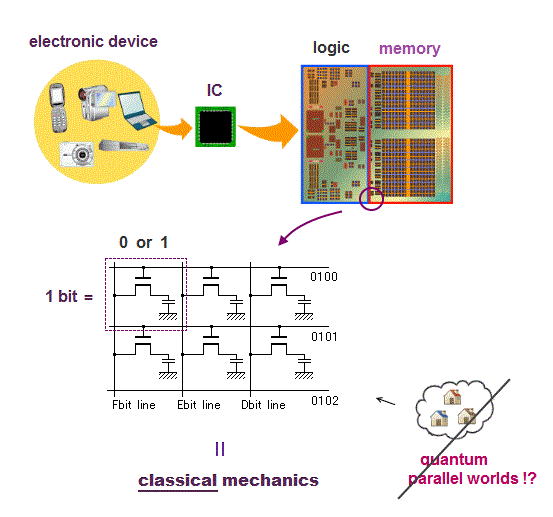"The current computer uses quantum mechanics ?" is untrue and fake news.

All electronic devices and computer CPU uses only classical mechanics ( Not quantum mechanics ! )

Computer is a collection of simple bits.

Each bit can express only two states on (= 1 ) or off (= 0 ) depending on whether charges are accumulated in a capacitor inside each bit.

This is purely classical mechanics, because a electron in quantum mechanics is just "vague electron cloud", Not a clear moving particle.

## PC transistor is classical mechanics.

### [ A electron in PC is a real moving particle, Not vague quantum wavefunction. ]

(Fig.2)  ↓ Charges accumulate in a capacitor or not = 1 or 0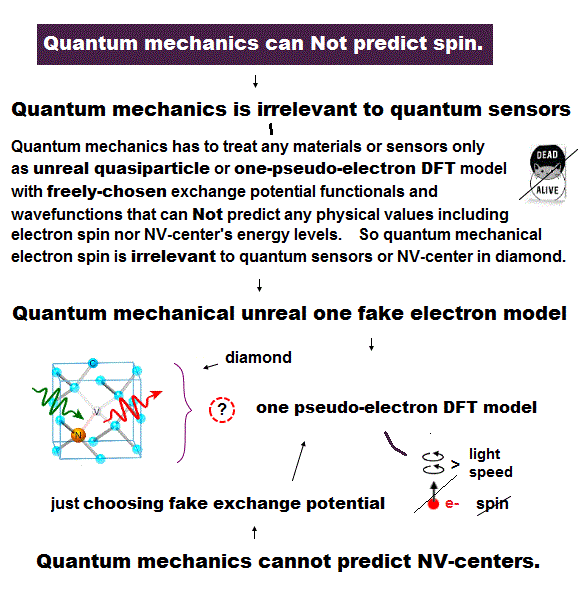The point is electrons (= charges ) inside PC do Not use Schrödinger equation or unreal spin of quantum mechanics at all !

PC is simple, some amount of electrons are moving and accumulate in a capacitor or not in each bit to express 1 or 0 state ( this p.9 ).

The most common type of "bit" is dynamic RAM, each bit consists of a pair of a transistor and a capacitor.

A transistor is made of special semiconductor, which allow electric current to flow or not depending on base (= gate ) voltage (= VG ).

When the voltage VG is on, electric current flows into (= 1 ) or out of (= 0 ) a capacitor in each bit.

That's all, so computers do not use quantum mechanics (= Schrödinger wavefunction or electron spin .. ) at all.

## The current computer is too big !

### [ Each bit still consists of many atoms.  ← Moore's law ends ? ]

(Fig.3)  ↓ The current computer is Not a "nano-machine".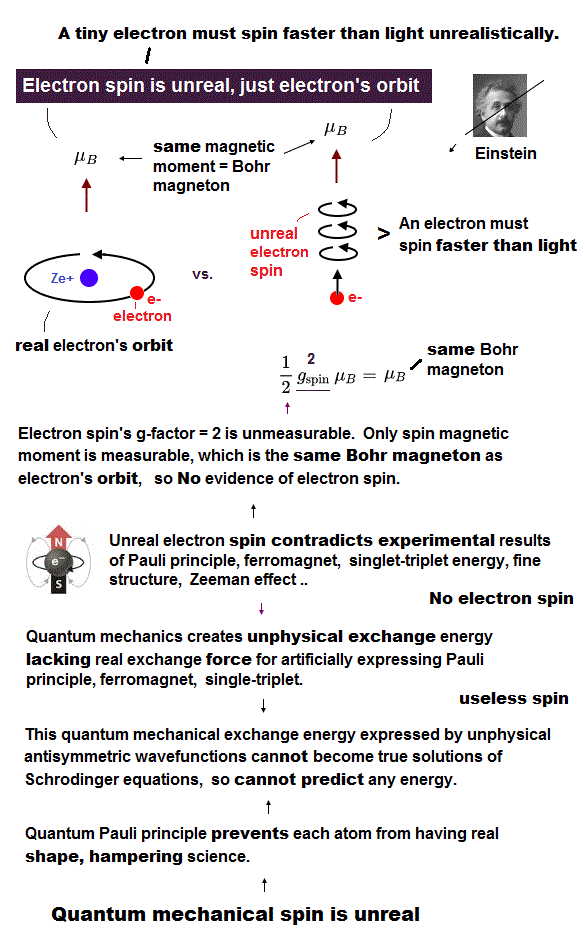Computer technology has rapidly progressed for the past decades, and PC becomes smaller and more energy-efficient.

But recently, this speed becomes slow, and Moore's law is dying.
We have already reached the limit of smaller computer size ?

In fact, the current computer is still very big !
The length of one side of each bit (= transistor ) is about 50nm.

Each atomic size is only 0.1nm, so a single bit in the current PC still contains a lot of atoms (= about 100 million atoms ! ).

If we can manipulate and use a single atom as a bit, we can drastically reduce the computer size to 1/100 million of the current PC  → nano-machine !

The current computer IC is made by photolithography and several chemical reactions, Not by manipulating each atom, though we already can manipulate an atom.

## We need to know 'electrons'.

### [ Electrons' orbital radius and motion are needed to manipulate an atom. ]

(Fig.4)  ↓ "Real" moving electrons and orbital radius → estimate force !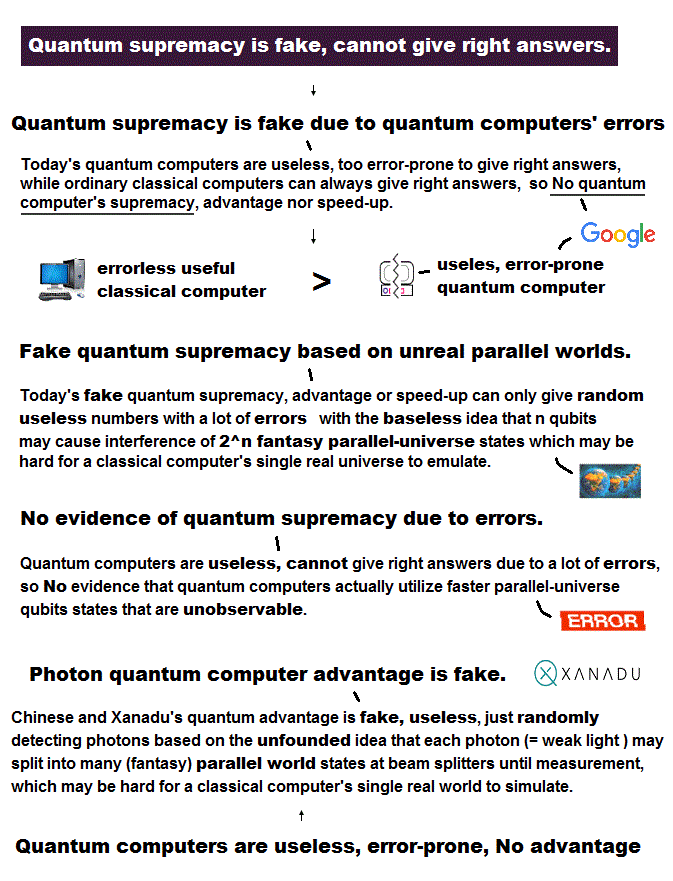We alredy have technology of manipulating a single atom, but old useless quantum mechanics prevents us from advancing further.

To manipulate each atom, we have to identify each atom and estimate their atomic force.

To estimate atomic Coulomb force, we need to treat each electron as a "real separated moving particle", and need to know their orbital radius.

But old quantum mechanics can only give vague electron cloud wavefunction, it can not use a real separated electron particle or "force".

Multi-electron atoms have No solution in Schrödinger equation.
It approximately uses hydrogen wavefunction in other atoms, though it's a fake solution.

We have to use de Broglie wavelength to estimate each atomic radius.
But quantum mechanics does not accept clear electron's orbit or de Broglie wave, so it cannot estimate clear orbital radius.

This is why old quantum mechanics is useless when we try to manipulate and identify a single atom, and it has to rely on unreal concepts in solid-state physics.

## "Real" atomic structure.

### [ Real electrons are serarated, moving, avoiding each other. ]

(Fig.5)  ↓ Electrons are moving, avoiding each other, Not electron cloud.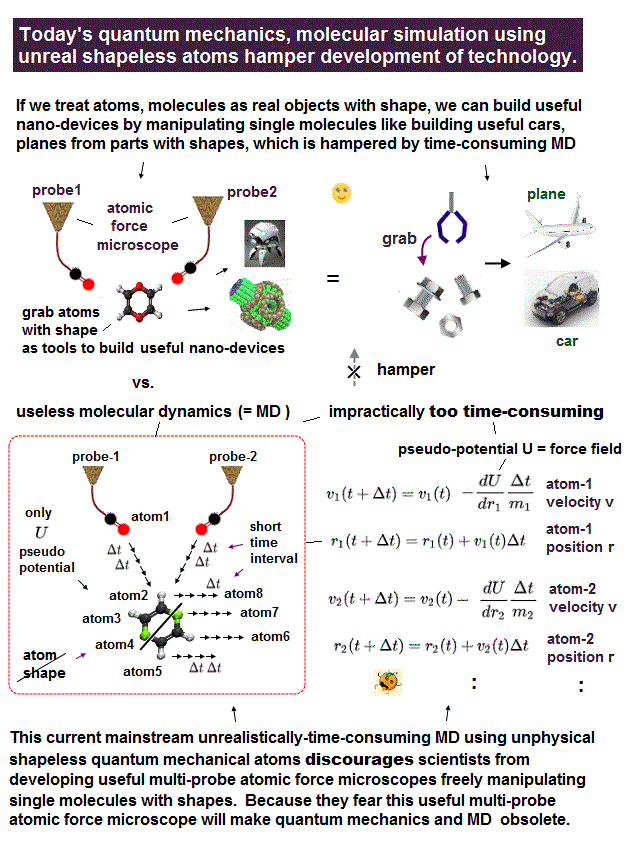To estimate Coulomb attraction between atoms, we need to know orbital radius and treat each electron as a moving particle avoiding each other.

Quantum mechanics only gives vague electron cloud (= Not a separated particle ).

Quantum wavefunctions don't have clear separated electrons or their orbital radius, so Schrödinger electrons cannot avoid each other (= always "overlap" ).

Quantum mechanics just chooses approximate (= fake ) solutions.

Carbon and silicon have the same number (= four ) of valence electrons, but their orbital radii are different, which is seen in different bond lengths between C-C and Si-Si.

Using an integer times de Brolgie wavelength and total energy ( from total ionizing energies ), we can estimate each electron orbital radius, which agrees with experimental bond length.

In this way, we can estimate approximate Couloumb force between various atoms using simple real atomic structure, and apply them to more complicated atoms or molecules.

## Quantum "vague" electron cloud is useless.

### [ Quantum mechanics doesn't have electrons or orbital radius. ]

(Fig.6)  ↓ No clear electrons in quantum wavefunction.Electron cloud (= wavefunction ) in quantum mechanics does Not have clear moving (= avoiding ) electrons or their orbital radius, so it cannot estimate forces among atoms.

Electrons in Schrödinger atoms can exist everywhere, which means electrons don't avoid each other, differenet electrons can approach and overlap freely (= toral energy is Not conserved ).

This is why Schrödinger's multi-electron atoms have No solutions.  Ground state energy must be the single lowest energy due to energy conservation.

Pauli exclusion principle disagrees with electron spin.

In antisymmetric wavefunction, when two orbitals are the same, total wavefunction is cancelled to be zero ?  ← Pauli principle ?

Unfortunately, this artificial, nonphysical "antisymmetric" wavefunction cannot explain Pauli principle, either.

When we normalize it (= numerator divided by demomintor ), canceled wavefunction is recovered (ex. zero/zero = 1 ), so "cancelled antisymmetric wavefunction" is Not canceled to be zero, so meaningless.

For example, in quantum lithium, we can use slightly different 1s wavefunction from other two 1s wavefunctions instead of the 3rd 2s orbital in approximate variational method + antisymmetric trial functions, which gives lower energy than experimental value.

So quantum mechanical approximate method in multi-electron atoms fails.

We have to consider de Broglie waves' interference and their collision for Pauli principle (= we can determine these de Broglie wave effect from experimental values, and apply it to more complicated atoms ).

## Solid physics in quantum mechanics is unreal.

### [ Fake electron, quasiparticle with fake mass in metal ? ]

(Fig.7)  ↓ Quantum mechanics lacks reality.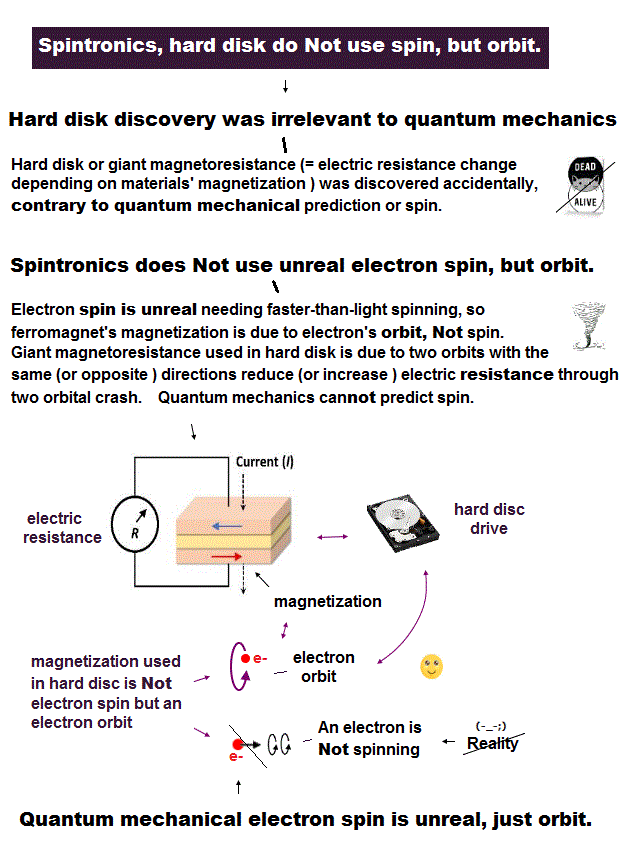Schrödinger equation is useless, then, how does quantum mechanics handle solid-state physics with muli-electrons ?

Surprisingly, they treat the whole solid as if it were a single fictitious quasi-electron with fake (= effective ) mass, which can be negative or changed in different directions !

In this "fictitious" quantum mechanics, all concepts such as mass, pseudo-spin, momentum are illusion ( this p.12 ).

Quantum mechanics has to rely on many unreal quasiparticles with fake mass to explain various phenomena, so wrong.

## Why science stops advancing ?

### [ Technology is great,  but theory is wrong. ]

(Fig.8)   The current technology can manipulate a single atom.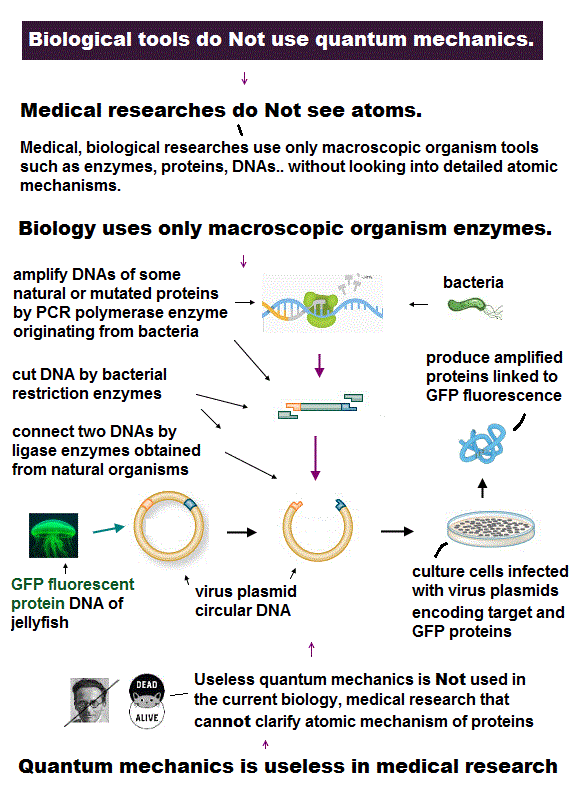We already have technology of manipulating a single atom.

So we should be able to make and manipulate tiny molecular machine to cure fatal disease.  But we cannot

Because the current basic physics is useless and discarded real atomic picture.

We need only two basic physical conditions to predict all atomic behaviors with the help of modern computers.

If there is only Coulomb-force condition, atomic (elecron orbital) radius is not determined.

de Broglie wavelength determines atomic size, and it can explain the different sizes between carbon and silicon.

These two conditions can explain the maximum electrons' number (= Pauli principle ) correctly, without unreal spin.

This simple atomic model (= only Coulomb + de Broglie ) can be easily applied to much bigger, complicated molecules with the help of computer to cure fatal disease.

So the first thing we should do now is discard the current old useless theories, and adopt more realistic ones to control each single atom for future nanomachines.2018/10/29 updated. Feel free to link to this site.